# Chapter 9 Chemical Reactions 9 1 Reactions Equations

• Slides: 23Chapter 9 Chemical Reactions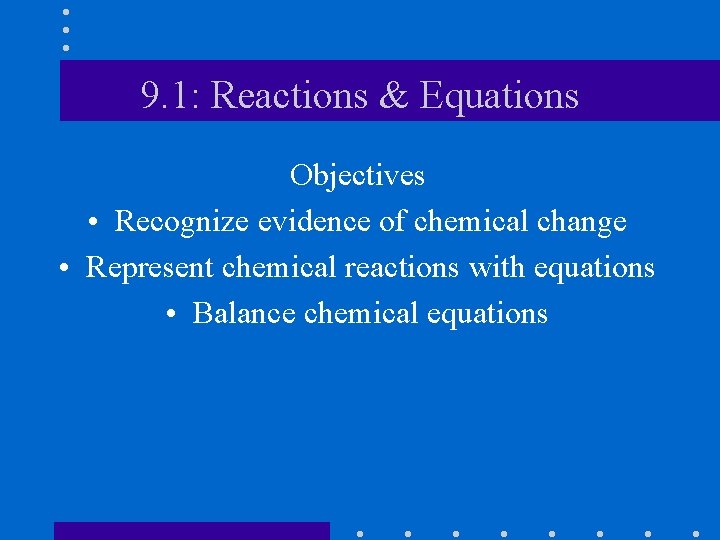9. 1: Reactions & Equations Objectives • Recognize evidence of chemical change • Represent chemical reactions with equations • Balance chemical equations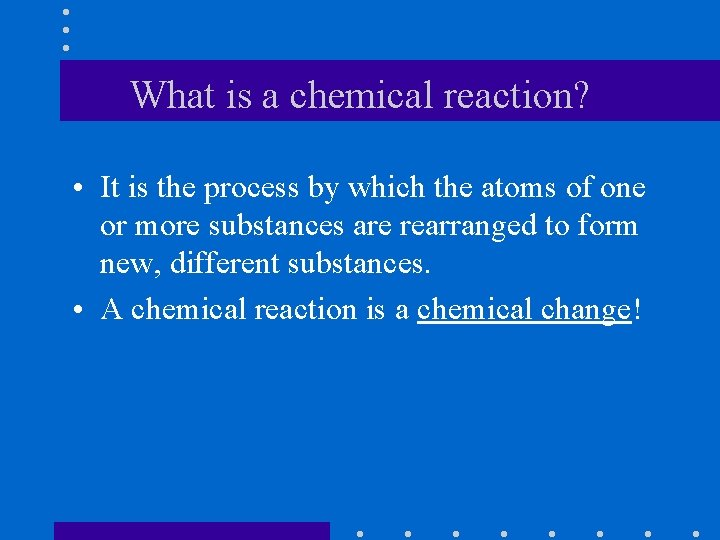What is a chemical reaction? • It is the process by which the atoms of one or more substances are rearranged to form new, different substances. • A chemical reaction is a chemical change!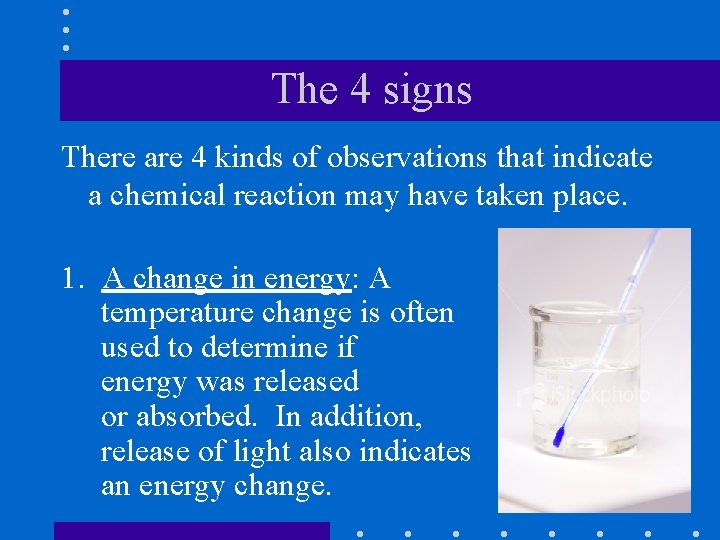The 4 signs There are 4 kinds of observations that indicate a chemical reaction may have taken place. 1. A change in energy: A temperature change is often used to determine if energy was released or absorbed. In addition, release of light also indicates an energy change.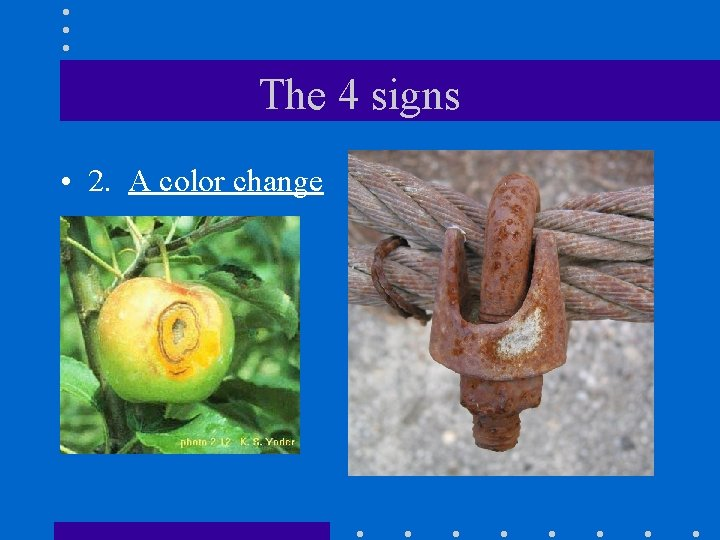The 4 signs • 2. A color change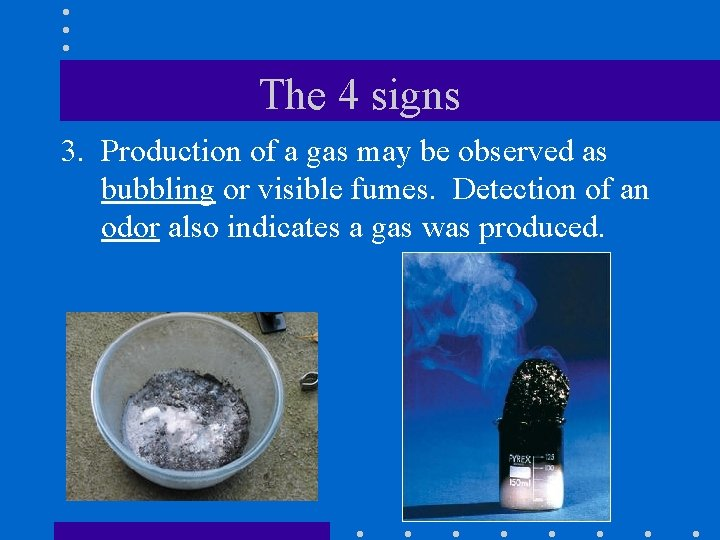The 4 signs 3. Production of a gas may be observed as bubbling or visible fumes. Detection of an odor also indicates a gas was produced.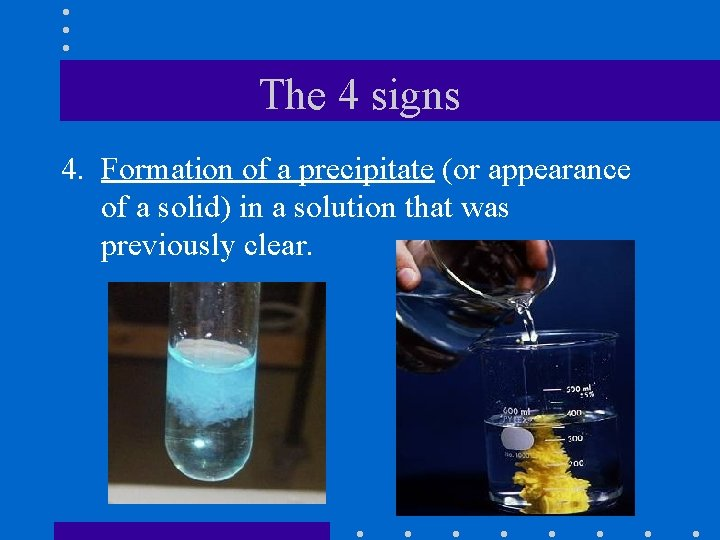The 4 signs 4. Formation of a precipitate (or appearance of a solid) in a solution that was previously clear.Practice • Recall burning magnesium as part of a lab activity. What evidence was there that a chemical reaction occurred?Review • Equations represent chemical reactions. • Reactants (on the left) are the starting substances. • Products (on the right) are the substances formed during the reaction.Review • An arrow shows the way the reaction progresses; It is found between the reactants and products and is usually read as “yields”. • “+” signs are placed between each reactant and product; They are read as “and”.Review • Symbols are often used after each reactant and product to indicate their state. – (s) for solid – (l) for liquid - (g) for gas - (aq) for water solutionReview • Coefficients are whole numbers written in front of formulas in the equation. They indicate the number of atoms, molecules, or ions that substance are involved in the reaction. of thatPractice • Identify the reactants and products and, using words not formulas, interpret the following equations. § Mg (s) + H 2 SO 4 (aq) H 2 (g) + Mg. SO 4 (aq) § C(s) + 2 Zn. O (s) 2 Zn (s) + CO 2 (g)Types of Equations • Word Equation: words are used to summarize the reaction. – Ex. : iron + chlorine – Ex. : carbon + sulfur iron (III) chloride carbon disulfide • Skeleton equation: Chemical formulas are substituted for words. – Ex. : Fe + Cl 2 – Ex. : C + S Fe. Cl 3 CS 2Skeleton Equations • Skeleton equations are also called unbalanced equations because they do not obey the Law of Conservation of Mass. – Fe + Cl 2 + Fe. Cl 3 1 iron atom 2 chlorine atoms 1 iron atom 3 chlorine atoms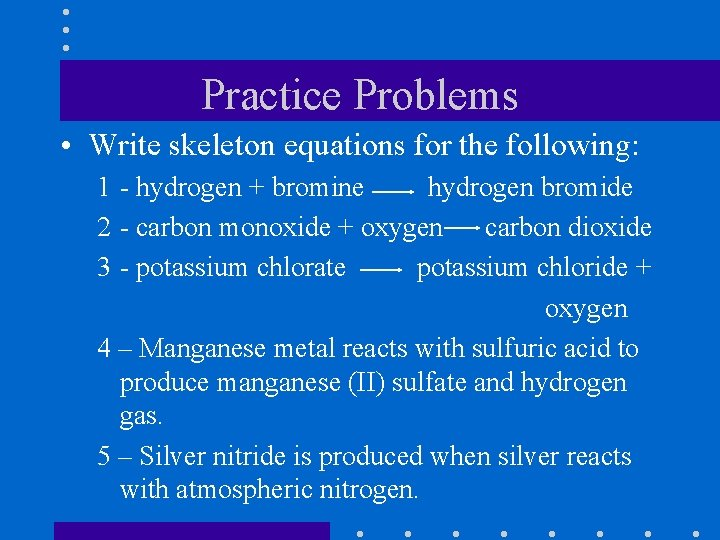Practice Problems • Write skeleton equations for the following: 1 - hydrogen + bromine hydrogen bromide 2 - carbon monoxide + oxygen carbon dioxide 3 - potassium chlorate potassium chloride + oxygen 4 – Manganese metal reacts with sulfuric acid to produce manganese (II) sulfate and hydrogen gas. 5 – Silver nitride is produced when silver reacts with atmospheric nitrogen.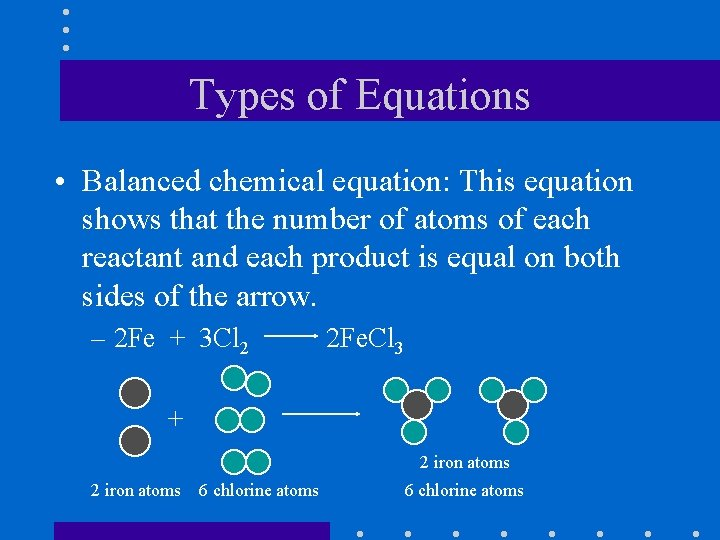Types of Equations • Balanced chemical equation: This equation shows that the number of atoms of each reactant and each product is equal on both sides of the arrow. – 2 Fe + 3 Cl 2 2 Fe. Cl 3 + 2 iron atoms 6 chlorine atoms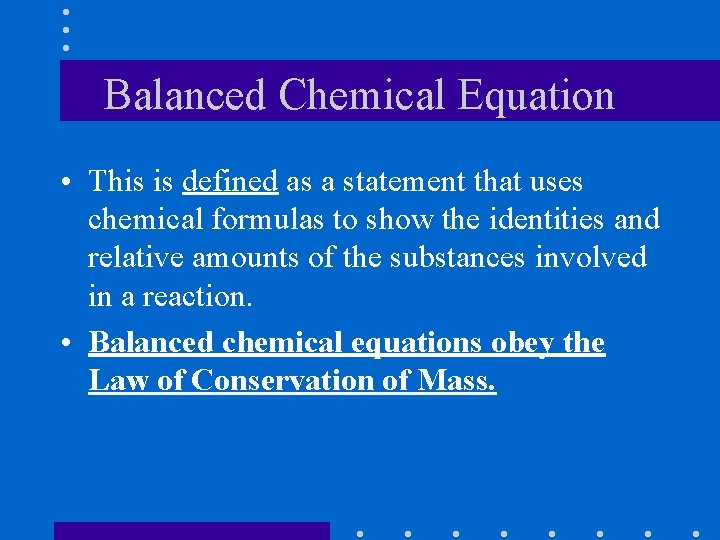Balanced Chemical Equation • This is defined as a statement that uses chemical formulas to show the identities and relative amounts of the substances involved in a reaction. • Balanced chemical equations obey the Law of Conservation of Mass.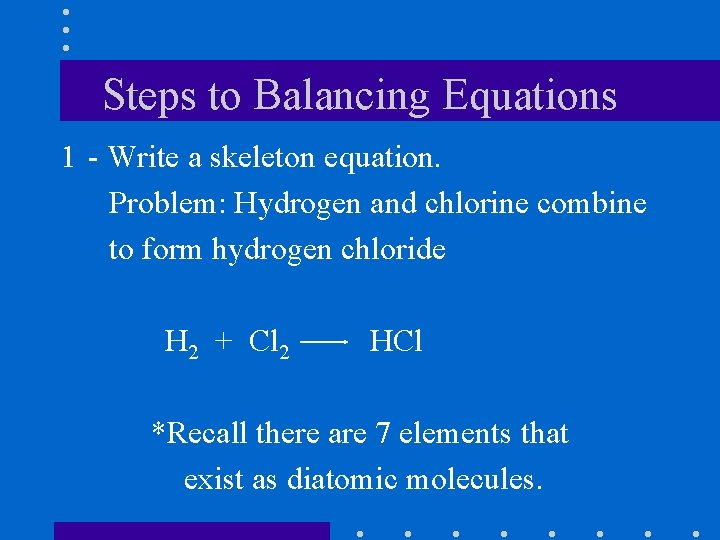Steps to Balancing Equations 1 - Write a skeleton equation. Problem: Hydrogen and chlorine combine to form hydrogen chloride H 2 + Cl 2 HCl *Recall there are 7 elements that exist as diatomic molecules.Steps to Balancing Equations 2 - Determine the number and kinds of atoms in the reactants. 3 - Determine the number and kinds of atoms in the products. H 2 + Cl 2 HCl 2 H 1 H 2 Cl 1 ClSteps to Balancing Equations 4 - Change the coefficients (never the subscripts) until the number of atoms of each element is equal on both sides of the equation. H 2 + Cl 2 2 HCl 2 H 2 1 H 2 Cl 2 1 ClSteps to Balancing Equations 5 - Make sure the coefficients are in the lowest possible ratio. Reduce if necessary. 6 - Go back and add the atoms up again. Make sure the number of atoms of each element is equal on both sides of the arrow. If you cannot balance the equation, go back and make sure your formulas are written correctly!!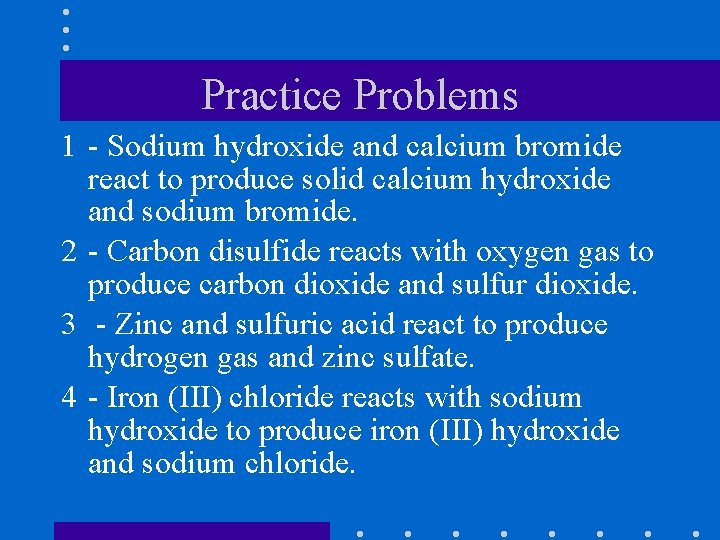Practice Problems 1 - Sodium hydroxide and calcium bromide react to produce solid calcium hydroxide and sodium bromide. 2 - Carbon disulfide reacts with oxygen gas to produce carbon dioxide and sulfur dioxide. 3 - Zinc and sulfuric acid react to produce hydrogen gas and zinc sulfate. 4 - Iron (III) chloride reacts with sodium hydroxide to produce iron (III) hydroxide and sodium chloride.